Friday, October 22, 2021
Home > IMO > Pre RMO Previous Year 2012 Question Paper With Solution

# Pre RMO Previous Year 2012 Question Paper With Solution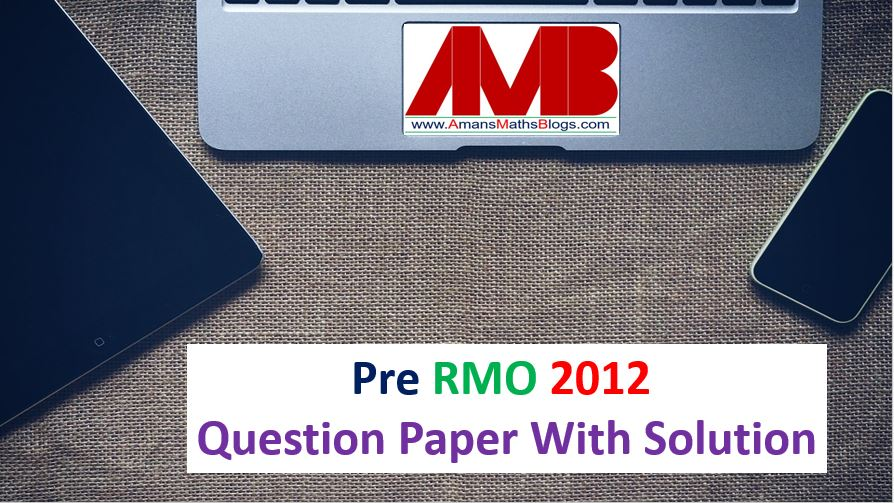# Pre RMO Previous Year 2012 Question Paper With Solutions

In this post, you will get Pre RMO Previous Year 2012 Question Paper With Solutions.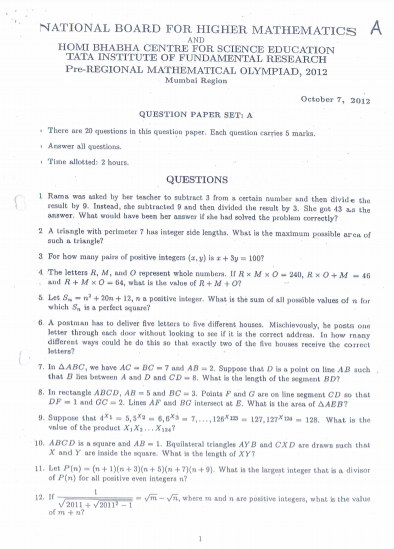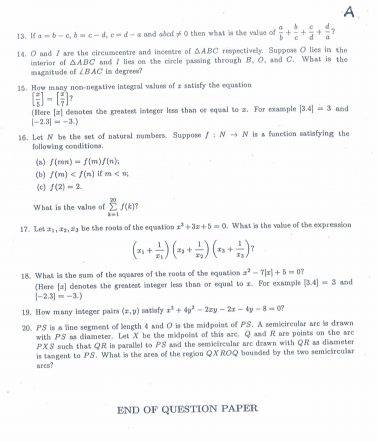Pre RMO Previous Year 2012 Question Paper Question No 1:

Rama was asked by her teacher to subtract 3 from a certain number and then divide the result by 9. Instead, she subtracted 9 and then divided the result by 3. She got 43 as the answer. What would have been her answer if she had solved the problem correctly?

Pre RMO Previous Year 2012 Solution of Question No 1: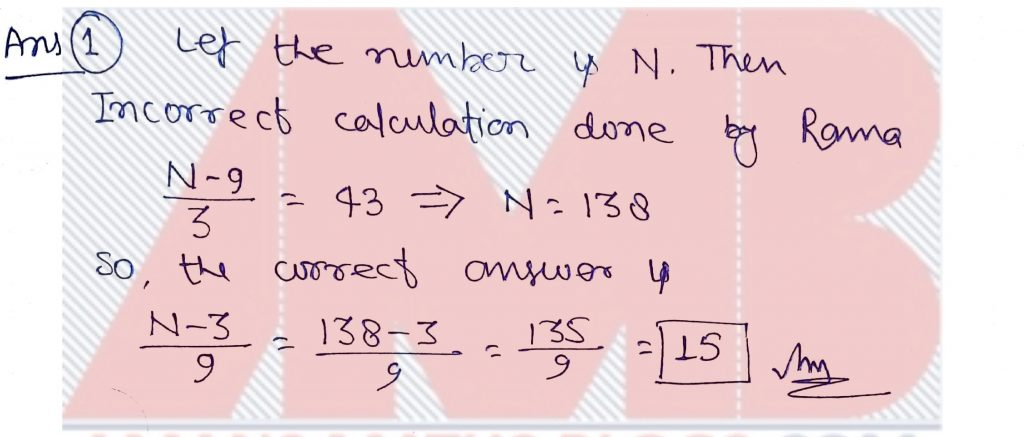Pre RMO Previous Year 2012 Question Paper Question No 2:

A triangle with perimeter 7 has integer side lengths. What is the maximum possible area of such a triangle?

Pre RMO Previous Year 2012 Solution of Question No 2: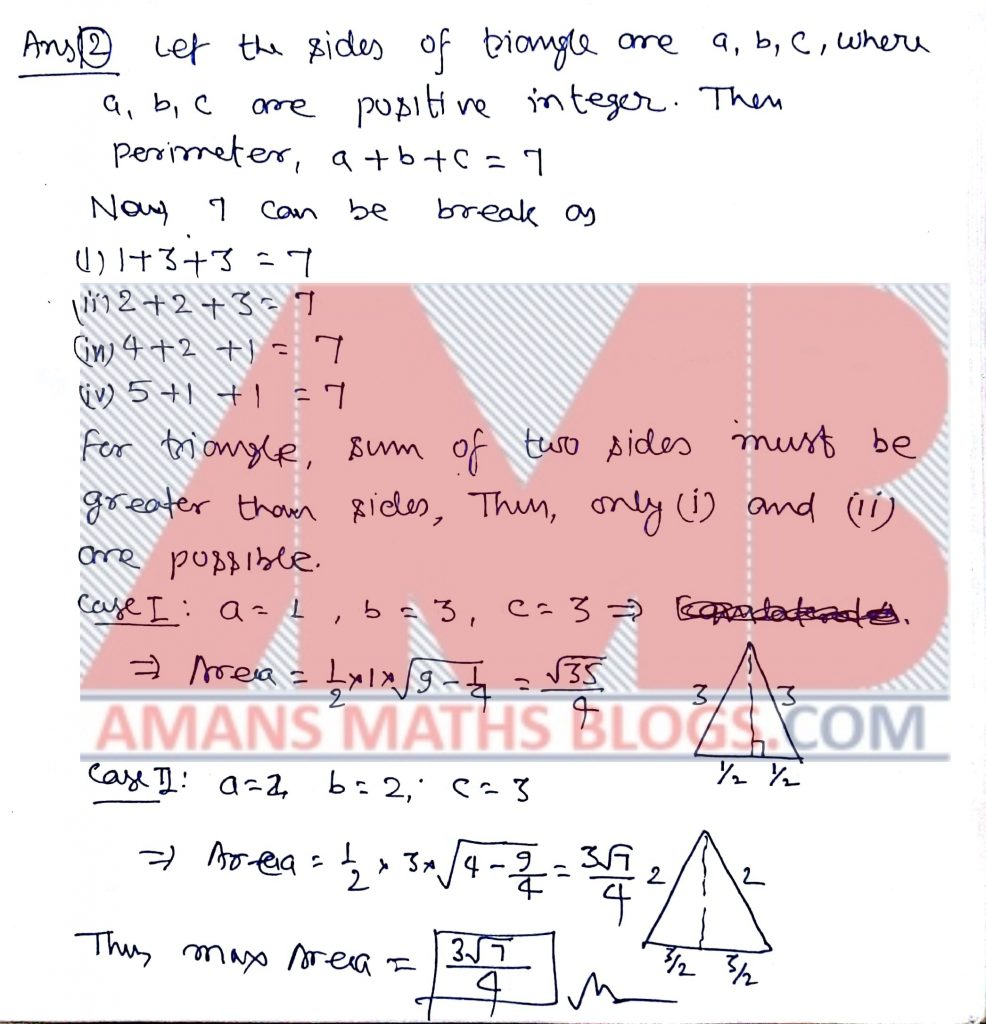Pre RMO Previous Year 2012 Question Paper Question No 3:

For how many pairs of positive integers (x, y) is x + 3y = 100?

Pre RMO Previous Year 2012 Solution of Question No 3: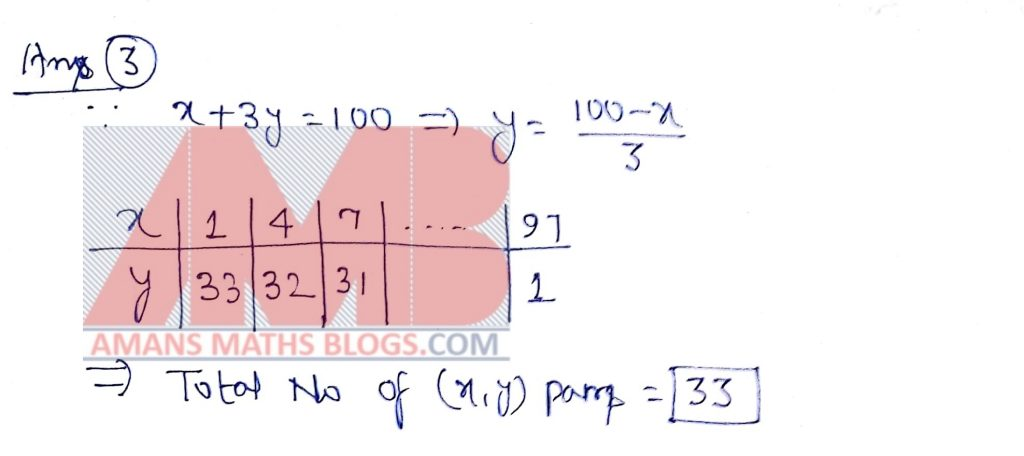Pre RMO Previous Year 2012 Question Paper Question No 4:

The letters R, M and O represent whole numbers. If R*M*0 = 240, R*O+M=46 and R + M * O =64, what is the value of R+M+O?

Pre RMO Previous Year 2012 Solution of Question No 4: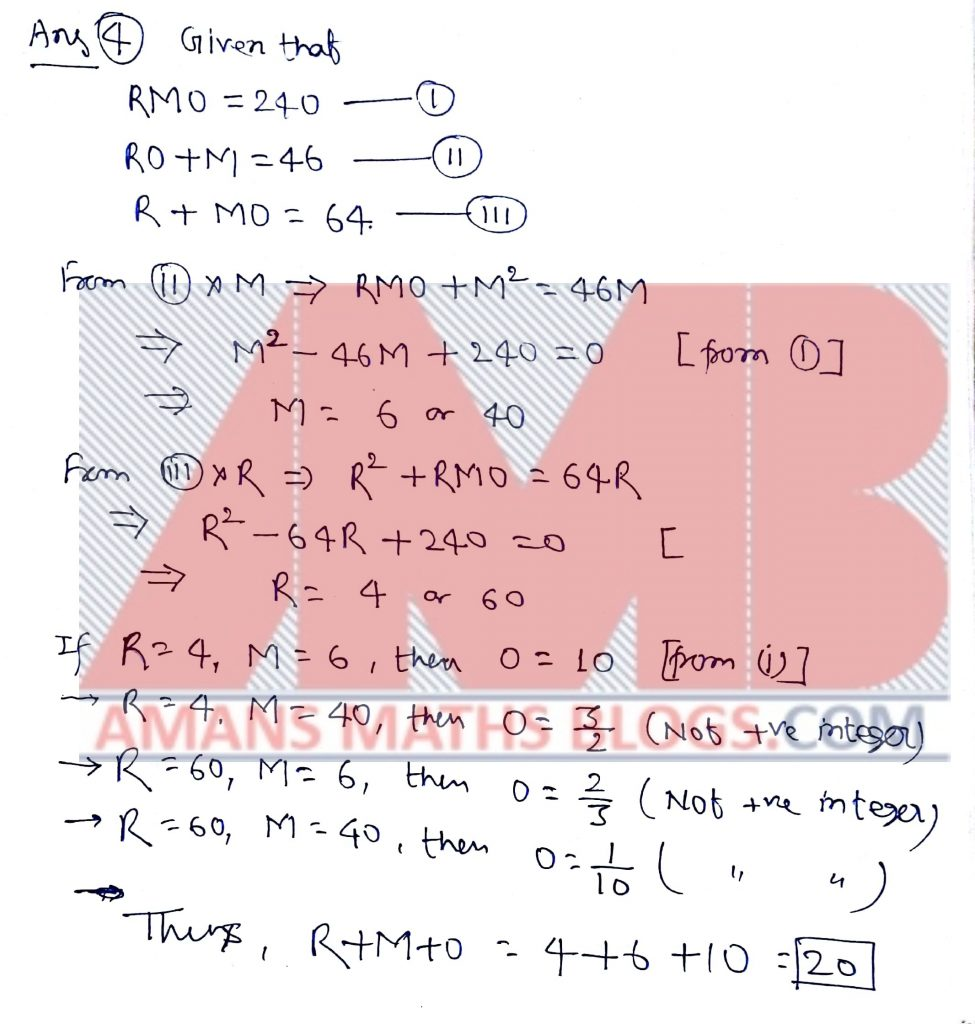Pre RMO Previous Year 2012 Question Paper Question No 5:

Let Sn = n+ 20n + 12, n a positive integer. What is the sum of all possible values of n for which Sn is a perfect square?

Pre RMO Previous Year 2012 Solution of Question No 5:Pre RMO Previous Year 2012 Question Paper Question No 6:

A Postman has to deliver five letters to five different houses. Mischievously, he posts one letter though each door he do this so that exactly two of the five housed receive the correct letters?

Pre RMO Previous Year 2012 Solution of Question No 6: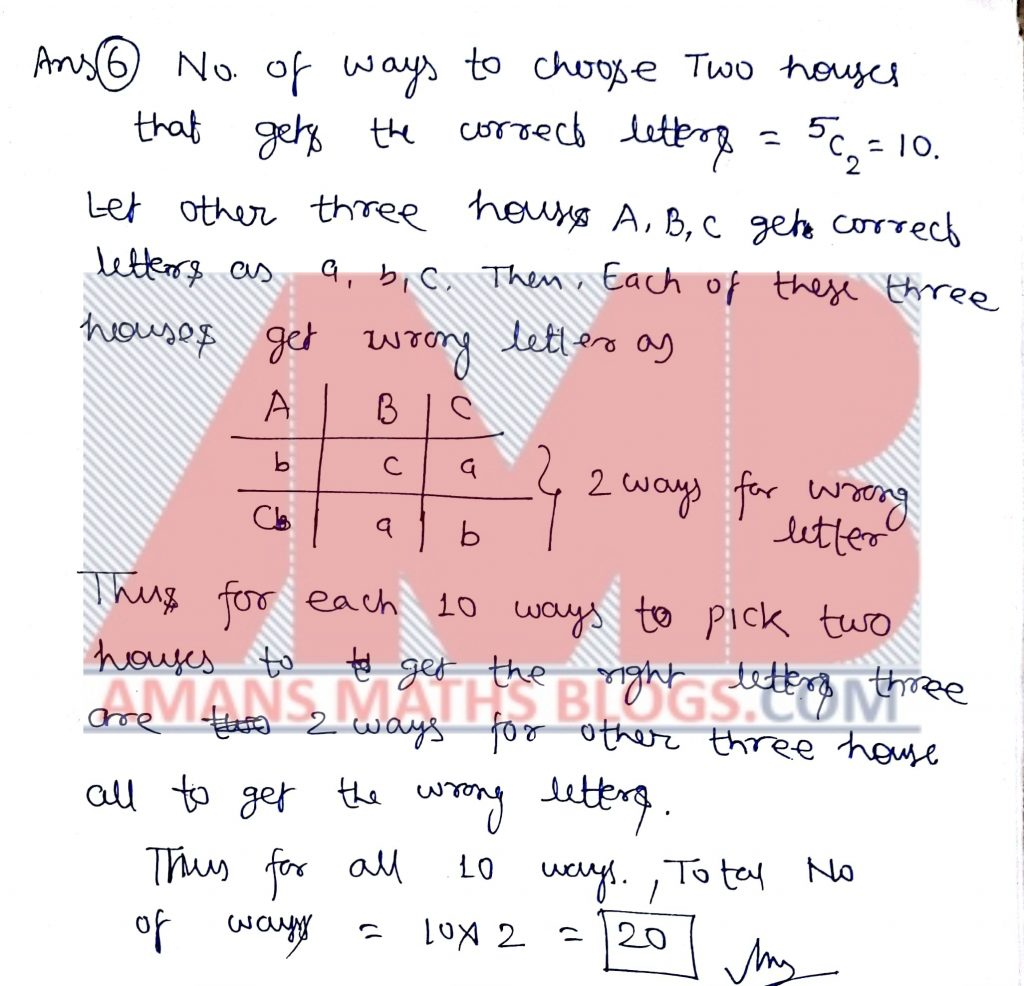Pre RMO Previous Year 2012 Question Paper Question No 7:

In ABC, we have AC = BC = 7 and AB = 2. Suppose that D is a point on line AB such that B lies between A and D and CD = 8. What is the length of the segment DB?

Pre RMO Previous Year 2012 Solution of Question No 7: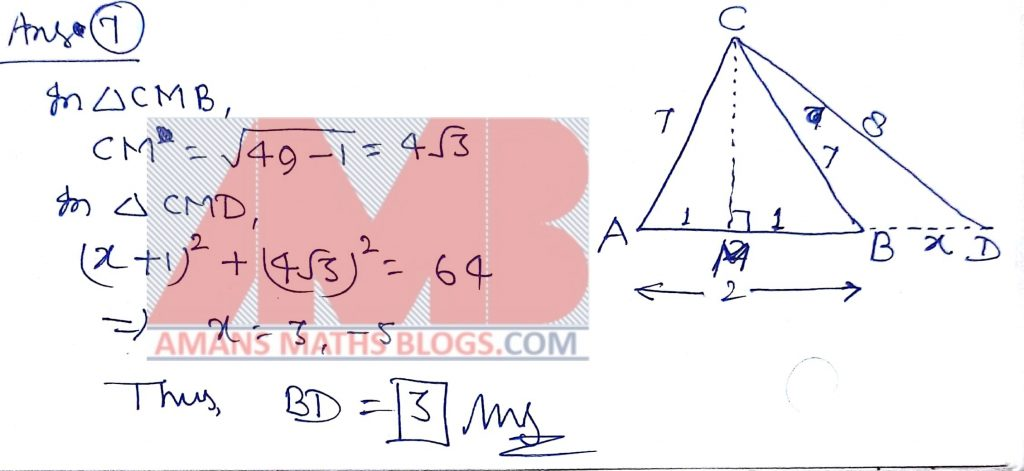Pre RMO Previous Year 2012 Question Paper Question No 8:

In rectangle ABCD, AB = 5 and BC = 3. Point F and G are on line segment CD so that DF = 1 and GC = 2. Lines AF and BG intersect at E. What is the area of AEB?

Pre RMO Previous Year 2012 Solution of Question No 8: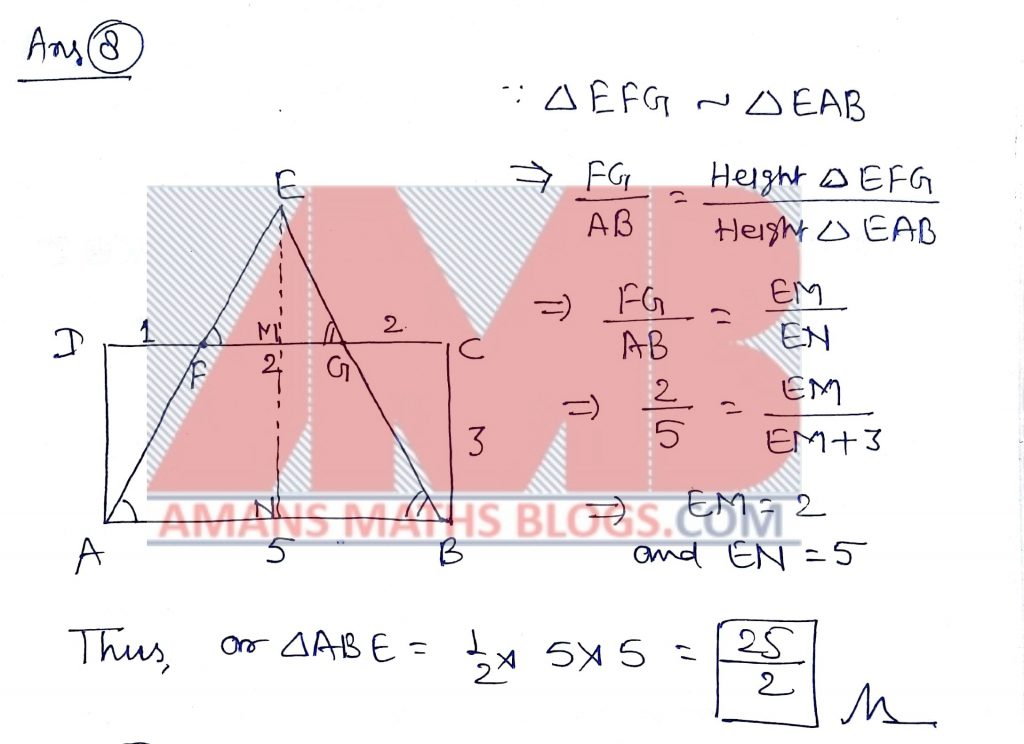Pre RMO Previous Year 2012 Question Paper Question No 9:

Suppose that 4x1 = 5, 5x2 = 6, 6x3 = 7 ,…, 123x123 = 127, 127x124 = 128. What is the value of the product x1 x2 . . . x124?

Pre RMO Previous Year 2012 Solution of Question No 9: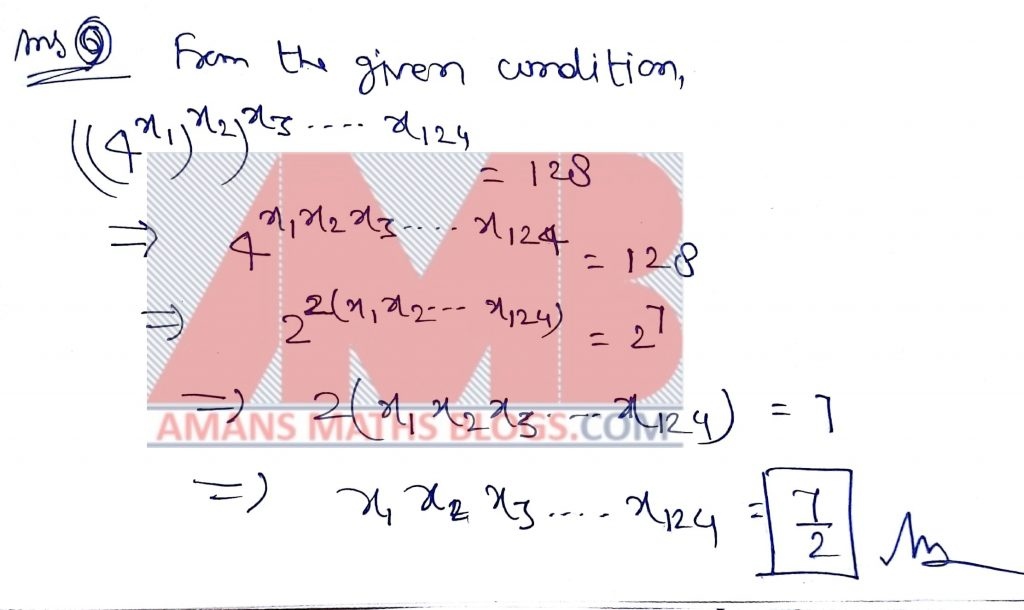Pre RMO Previous Year Question Paper Question No 10:

ABCD is a square and AB = 1. Equilateral triangles AY B and CXD are drawn such that X and y are inside the square. What us the length of XY?

Pre RMO Previous Year 2012 Solution of Question No 10: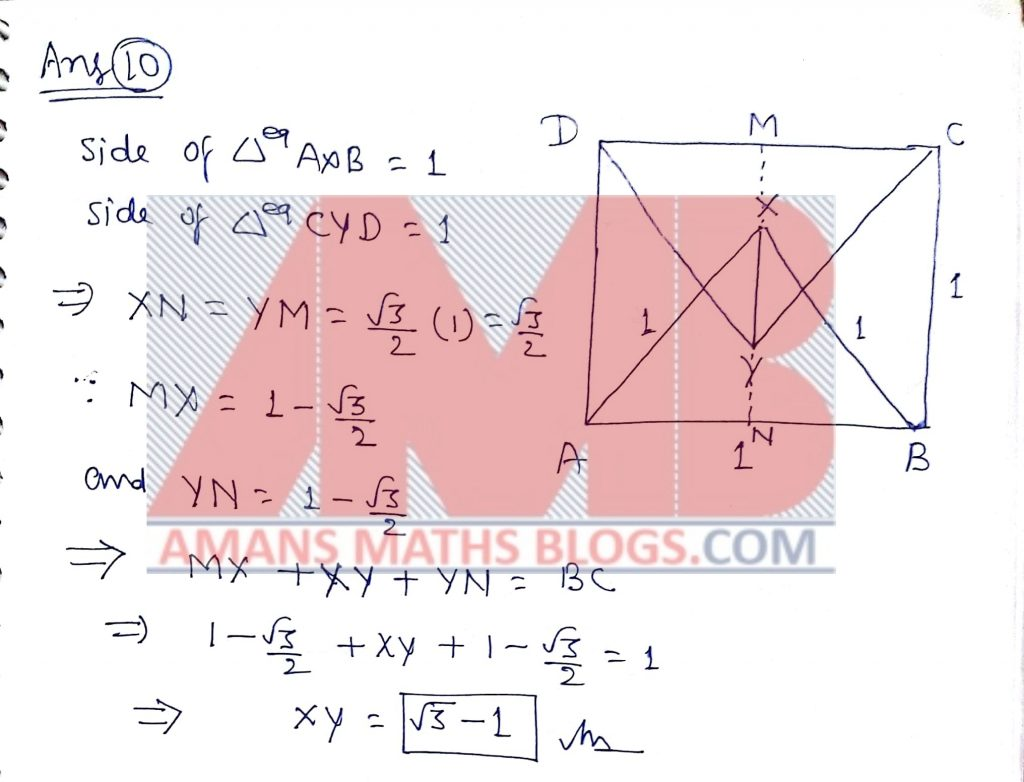Pre RMO Previous Year 2012 Question Paper Question No 11:

Let P(n) = (n+1)(n+3)(n+5)(n+7)(n+9). What is the largest integer that is a divisor of P(n) for all positive even integers n?

Pre RMO Previous Year 2012 Solution of Question No 11: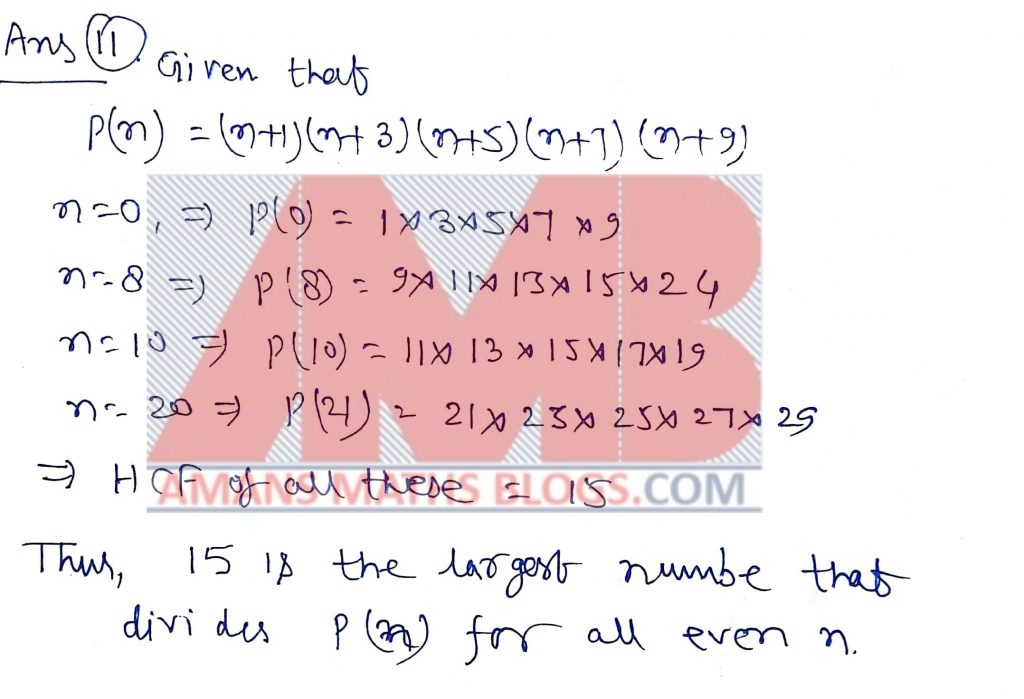Pre RMO Previous Year Question Paper Question No 12:

Ifwhere m and n are positive integers, what is the value m + n.

Pre RMO Previous Year 2012 Solution of Question No 12: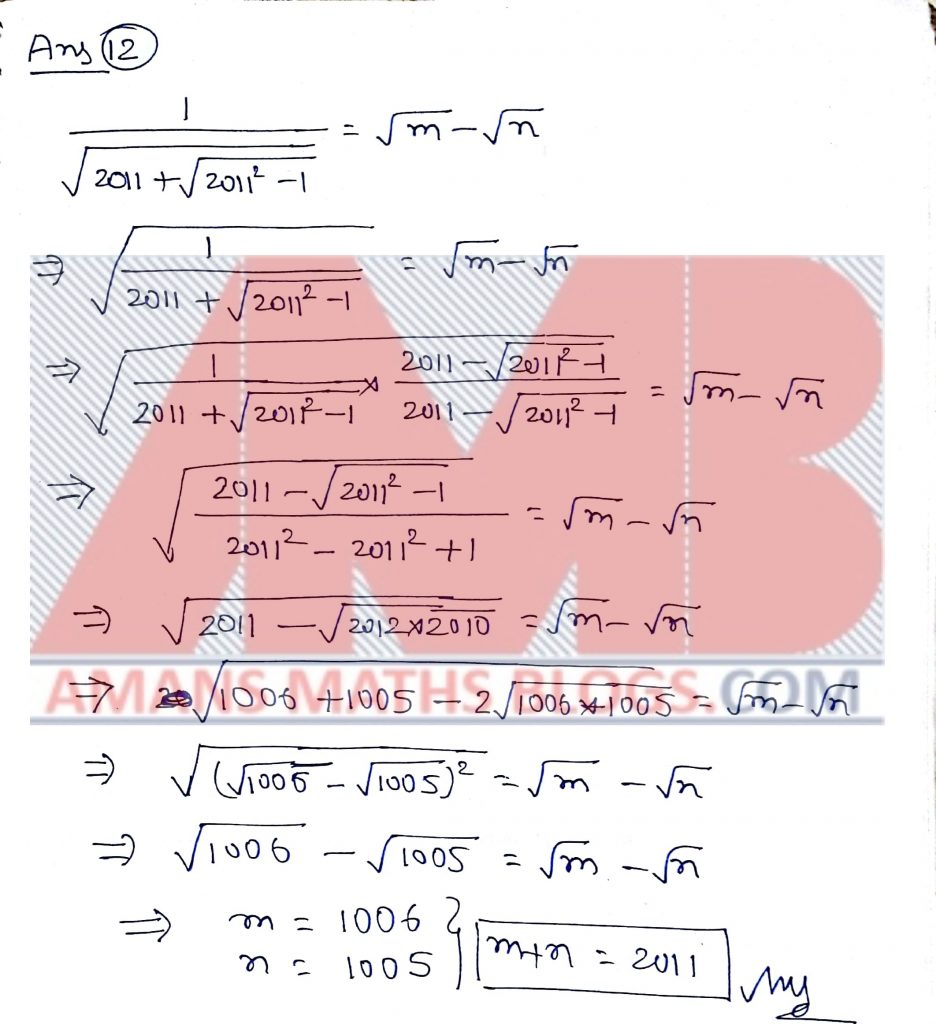Pre RMO Previous Year 2012 Question Paper Question No 13:

If a =b – c, b=c – d, c= d – a and abcd0 then what is the value of a/b + b/c + c/d + d/a?

Pre RMO Previous Year 2012 Solution of Question No 13: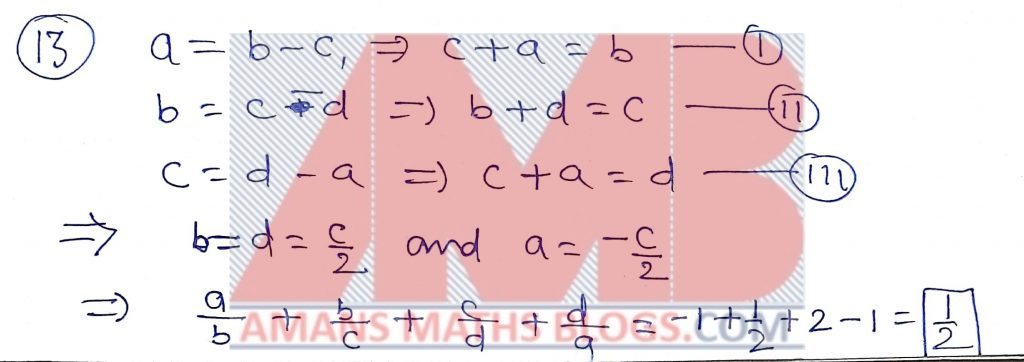Pre RMO Previous Year Question Paper Question No 14:

O and I are the circumcenter and incentre of ABC respectively. Suppose O lines in the interior of triangle ABC and I lines on the circle passing through B, O, and C. What is the magnitude of angle BAC in degrees?

Pre RMO Previous Year 2012 Solution of Question No 14: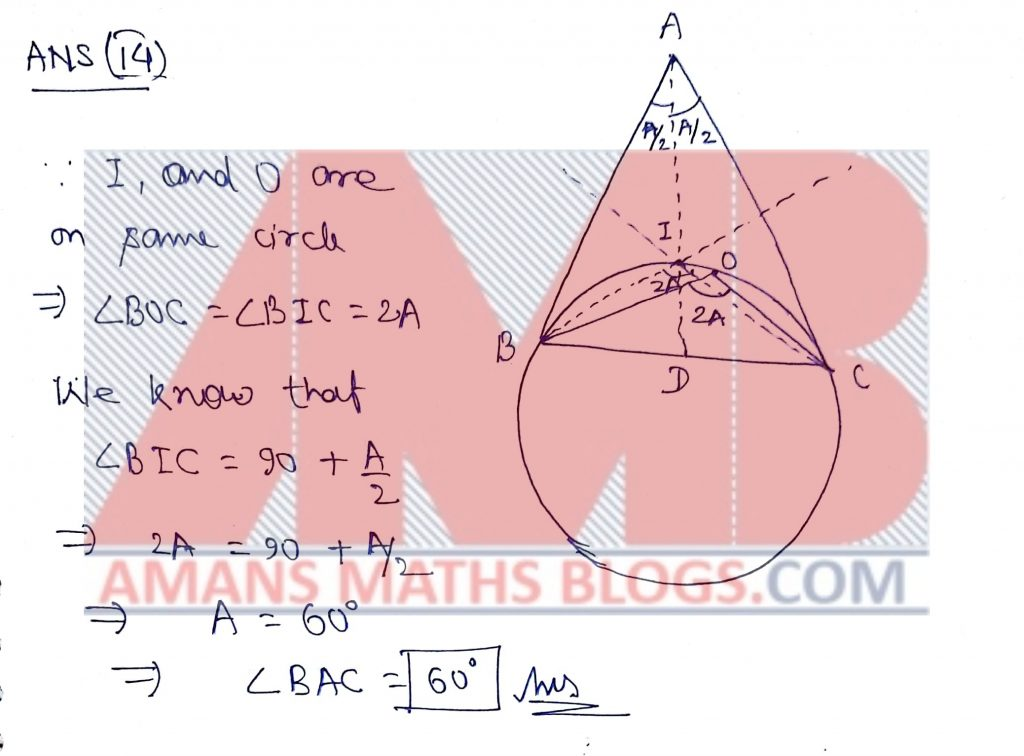Pre RMO Previous Year Question Paper Question No 15:

How many non-negative integral values of x satisfy the equation [x/5] = [x/7]?

(Here [x] denotes the greatest integer less than of equal to x. For example [3.4] = 3 and [-2.3] = -3.)

Pre RMO Previous Year 2012 Solution of Question No 15: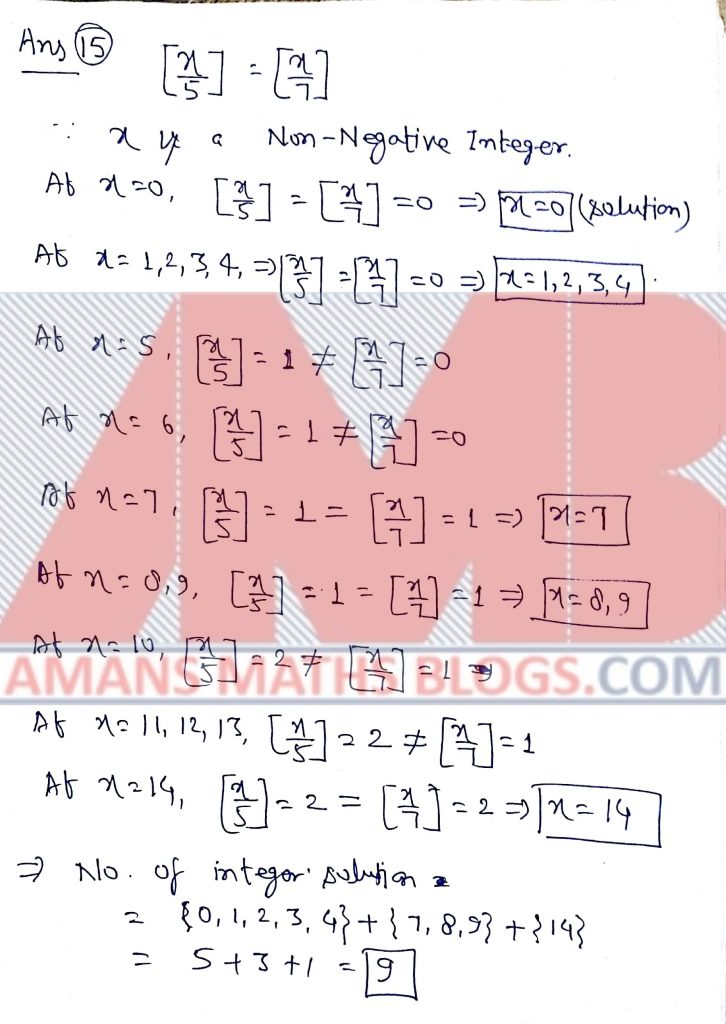Pre RMO Previous Year Question Paper Question No 16:

Let N be the set of natural numbers. Suppose f : NN is a function satisfying the following conditions.

• f(mn) = f(m)f(n);
• f(m) < f(n) if m < n;
• f(2) = 2.

What is the value of?

Pre RMO Previous Year 2012 Solution of Question No 16: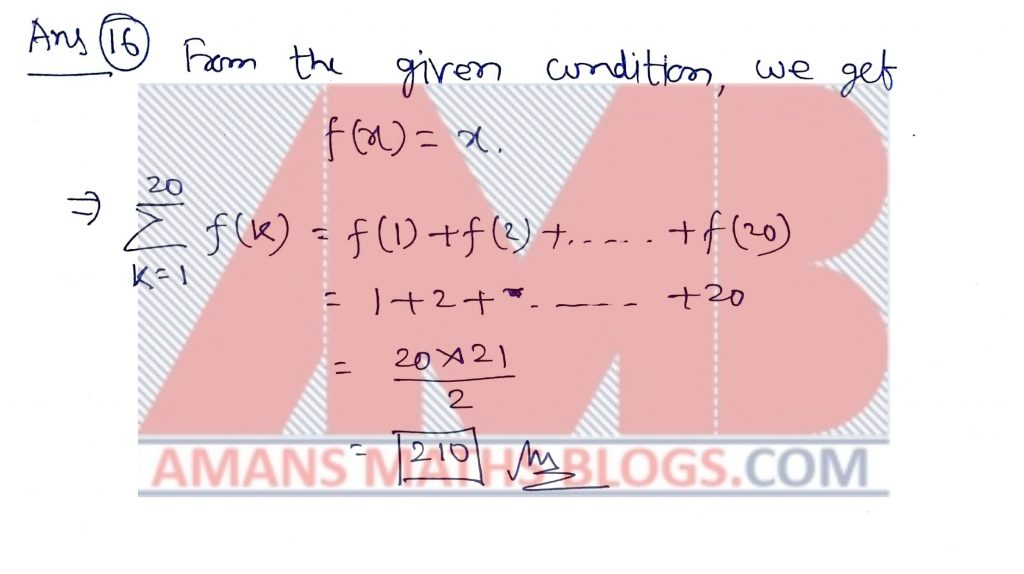Pre RMO Previous Year Question Paper Question No 17:

Let x1, x2, x3 be the roots of the equation x3 + 3x + 5 =0. What is the value of the expression.

Pre RMO Previous Year 2012 Solution of Question No 17: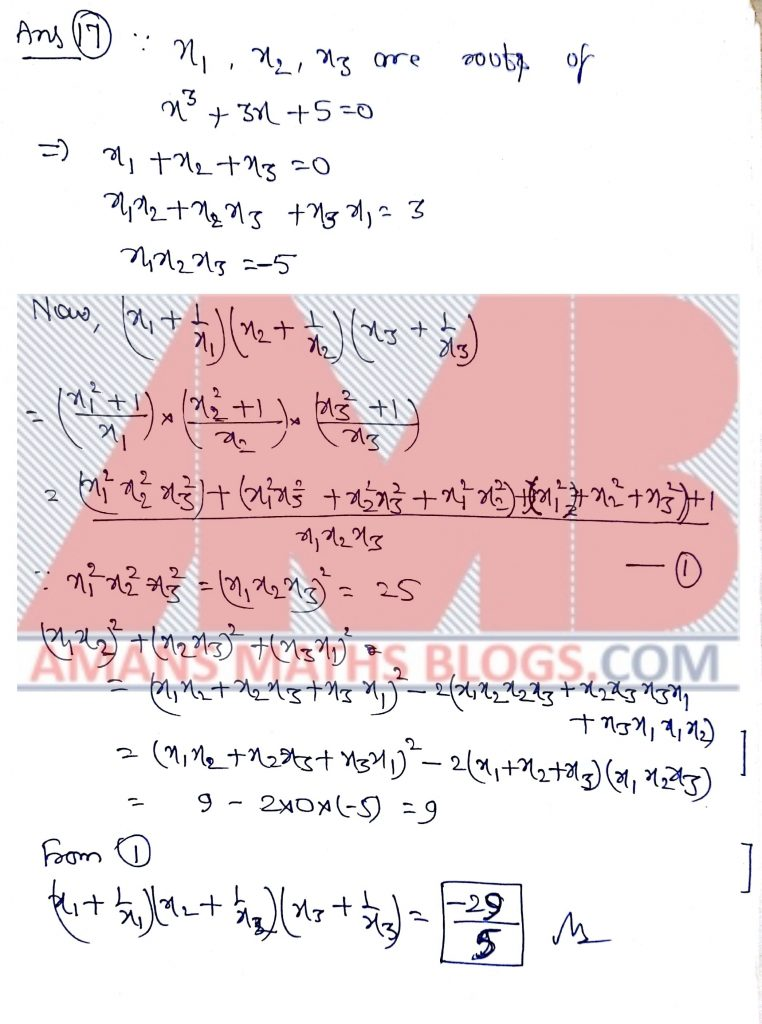Pre RMO Previous Year Question Paper Question No 18:

What is sum of the squares of the roots of equation x2 – 7[x] + 5 = 0? (Here [x] denotes the greatest integer less than or equal to x. For example [3.4] = 3 and [-2.3] = -3.)

Pre RMO Previous Year 2012 Solution of Question No 18: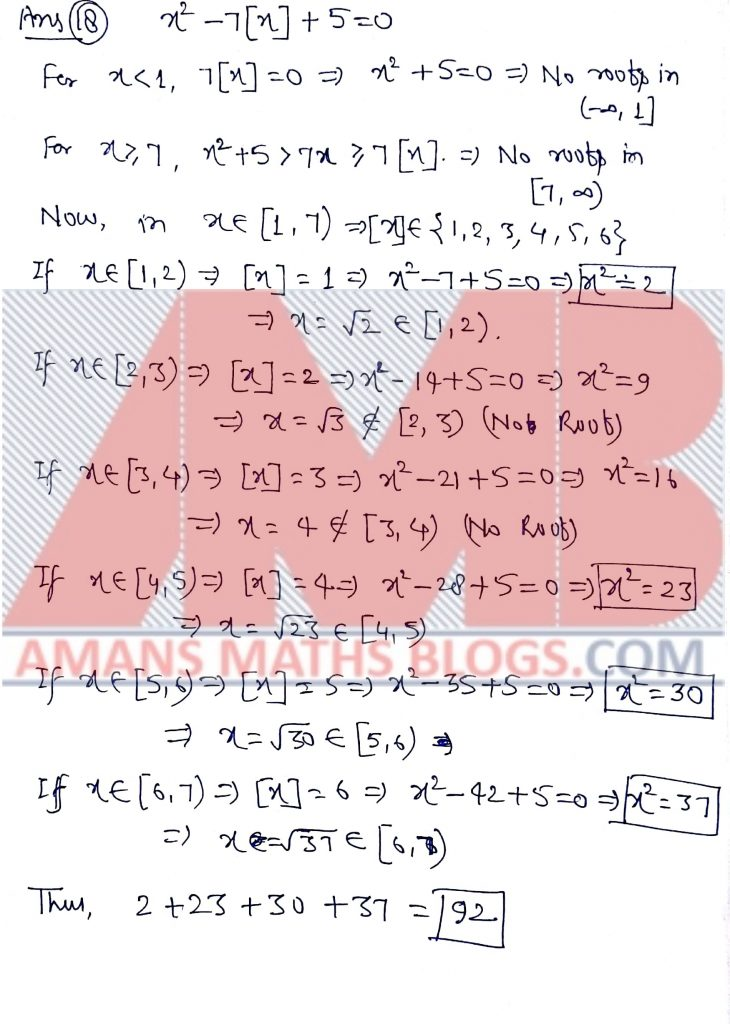Pre RMO Previous Year Question Paper Question No 19:

How many integers pairs (x, y) satisfy x2 + 4y2 – 2xy – 2x – 4y – 8 =0?

Pre RMO Previous Year 2012 Solution of Question No 19:Pre RMO Previous Year Question Paper Question No 20:

PS is a line segment of length 4 and O is the midpoint of PS. A semicircular are is drawn with PS as diameter. Let X be the midpoint of this arc. Q and R are points on the arc PXS such that QR is parallel to PS and the semicircular arc drawn with QR as diameter is tangent to PS. What is the area of the region QX ROQ bounded by the two semicircular arcs?

Pre RMO Previous Year 2012 Solution of Question No 20:Get Combined Pre RMO 2012 to 2018 Questions Paper with Solution (Click on Image)
AMBiPi
error: Content is protected !!